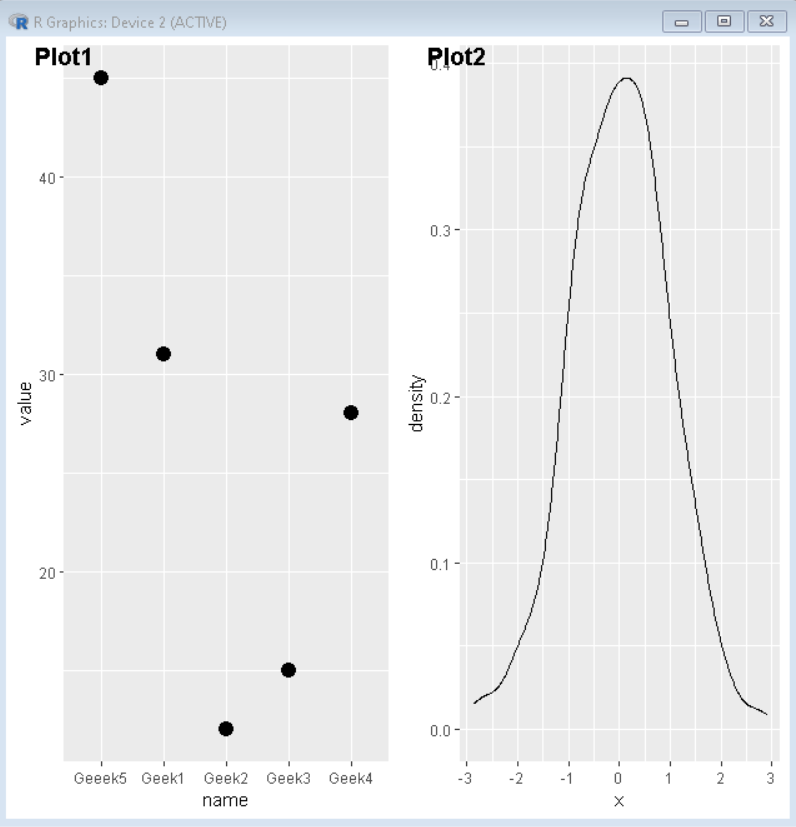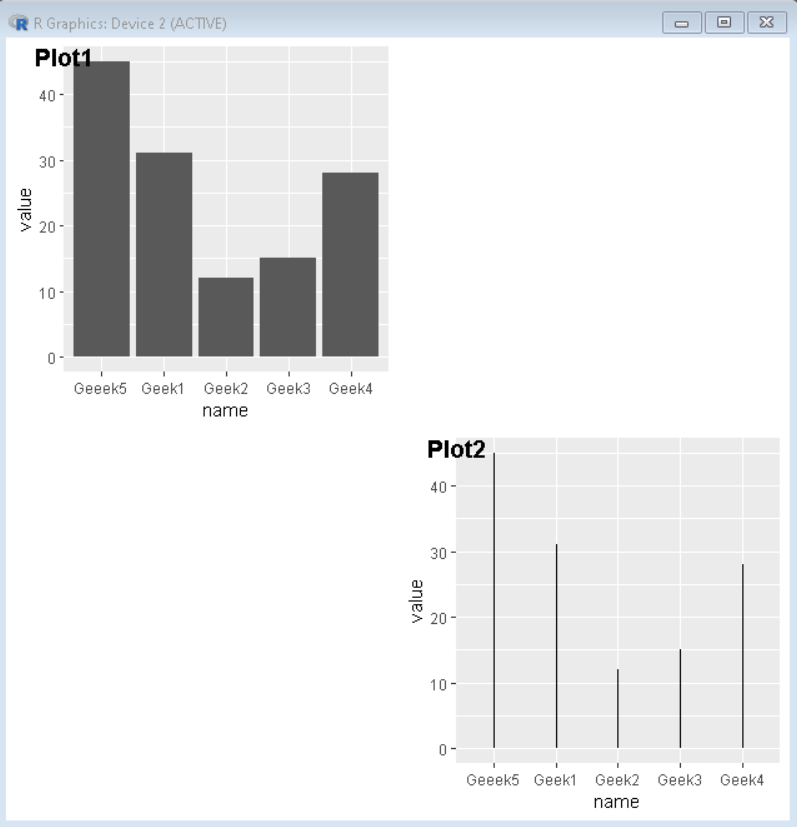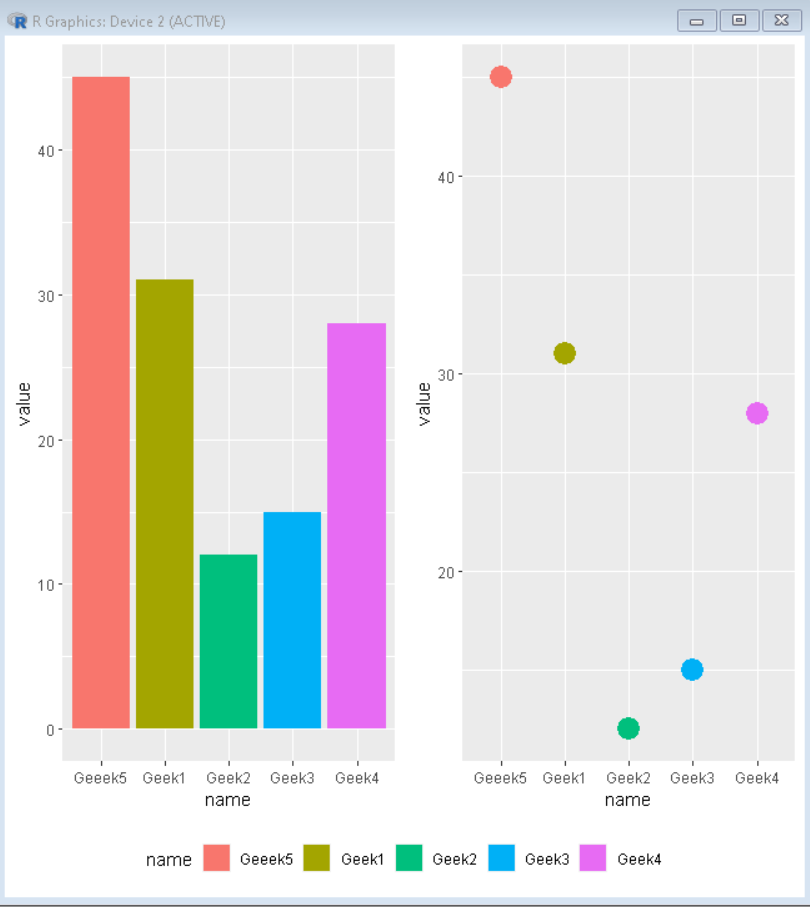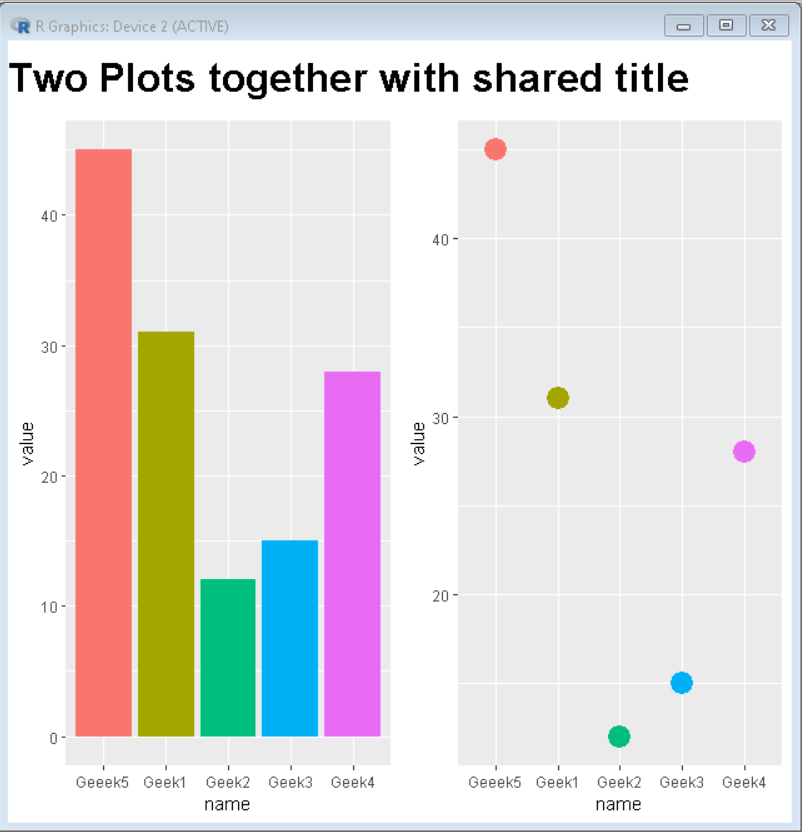GeeksforGeeks App
Open AppBrowser
Continue

# How To Join Multiple ggplot2 Plots with cowplot?

In this article, we are going to see how to join multiple ggplot2 plots with cowplot. To join multiple ggplot2 plots, we use the plot_grid() function of the cowplot package of R Language.

Syntax: plot_grid(plot1,plot2,label=<label-vector>, ncol, nrow)

Parameters:

• plot1 and plot2 are plots that need to be joined.
• <label-vector> is a vector that has a collection of labels for plots.
• ncol is the number of columns canvas will be divided in.
• nrow is the number of rows canvas will be divided in.

## R

 `# Create sample data``set.seed``(5642)                             ``sample_data1 <- ``data.frame``(``  ``name = ``c``(``"Geek1"``,``"Geek2"``,``"Geek3"``,``           ``"Geek4"``,``"Geeek5"``) ,``  ``value=``c``(31,12,15,28,45))`` ` `sample_data2 <- ``data.frame``(x = ``rnorm``(400))`` ` `# Load ggplot2 and cowplot``library``(``"ggplot2"``) ``library``(``"cowplot"``)`` ` `# Create both plot and store in variable``plot1<-``ggplot``(sample_data1,``              ``aes``(x = name, y = value)) +``geom_point``(size=4)`` ` `plot2<-``ggplot``(sample_data2, ``aes``(x = x)) +``geom_density``(alpha=0.8)``plot_grid``(plot1, plot2, labels = ``c``(``'Plot1'``, ``'Plot2'``))`

Output:Output

### Example 2: Create a gap between plots using NULL as plot value.

To fix the number of columns we use ncol attribute of plot_grid() function.

## R

 `# Create sample data``set.seed``(5642)                             ``sample_data <- ``data.frame``(``  ``name = ``c``(``"Geek1"``,``"Geek2"``,``"Geek3"``,``           ``"Geek4"``,``"Geeek5"``) ,``  ``value = ``c``(31,12,15,28,45))`` ` `# Load ggplot2 and cowplot``library``(``"ggplot2"``) ``library``(``"cowplot"``)`` ` `# Create both plot and store in variable``plot1<-``ggplot``(sample_data, ``aes``(x=name, y=value)) +``geom_bar``(stat = ``"identity"``)`` ` `plot2<-``ggplot``(sample_data, ``aes``(x = name, y=value)) +``geom_segment``( ``aes``(x=name, xend=name, y=0, yend=value)) `` ` `plot_grid``(plot1,``NULL``, ``NULL``, plot2, labels = ``c``(``  ``'Plot1'``,``''``,``''``, ``'Plot2'``), ncol=2)`

Output:Output

### Example 3: Side by Side plot with the shared legend.

To create a plot with a shared legend, we create plots without a legend and create a separate legend and combine them.

## R

 `# Create sample data``set.seed``(5642)                             ``sample_data <- ``data.frame``(name=``c``(``"Geek1"``,``"Geek2"``,``"Geek3"``,``                                 ``"Geek4"``,``"Geeek5"``) ,``                          ``value=``c``(31,12,15,28,45))`` ` `# Load ggplot2 and cowplot``library``(``"ggplot2"``) ``library``(``"cowplot"``)`` ` `# Create both plot and store in variable without legend``plot1<-``ggplot``(sample_data, ``aes``(x=name, y=value, fill=name)) +``geom_bar``(stat = ``"identity"``) +``theme``(legend.position = ``"none"``)`` ` `plot2<-``ggplot``(sample_data, ``aes``(x = name, y=value, fill=name)) +  ``geom_point``(``aes``(colour = ``factor``(name)), size = 6)+``theme``(legend.position = ``"none"``)`` ` `# combine both plot using plot_grid()``combined_plot<-``plot_grid``(plot1, plot2,ncol=2)`` ` `# extract legend from plot2``legend <- ``get_legend``(``  ``plot1 +``    ``guides``(color = ``guide_legend``(nrow = 1)) +``    ``theme``(legend.position = ``"bottom"``)``)`` ` `# Combine combined plot and legend using plot_grid()``plot_grid``(combined_plot, legend,ncol=1,rel_heights = ``c``(1, .1))`

Output:Output

### Example 4: Side by Side plot with a shared title

To create a plot with a shared title, we create plots without titles and create a separate title and combine them.

## R

 `# Create sample data``set.seed``(5642)                             ``sample_data <- ``data.frame``(name=``c``(``"Geek1"``,``"Geek2"``,``                                 ``"Geek3"``,``"Geek4"``,``"Geeek5"``) ,``                          ``value=``c``(31,12,15,28,45))`` ` `# Load ggplot2 and cowplot``library``(``"ggplot2"``) ``library``(``"cowplot"``)`` ` `# Create both plot and store in variable without legend``plot1<-``ggplot``(sample_data, ``aes``(x=name, y=value, fill=name)) +``geom_bar``(stat = ``"identity"``) +``theme``(legend.position = ``"none"``)`` ` `plot2<-``ggplot``(sample_data, ``aes``(x = name, y=value, fill=name)) +  ``geom_point``(``aes``(colour = ``factor``(name)), size = 6)+``theme``(legend.position = ``"none"``)`` ` `# combine both plot using plot_grid()``combined_plot<-``plot_grid``(plot1, plot2,ncol=2)`` ` `# create title for plot``title <- ``ggdraw``() + ``  ``draw_label``(``    ``"Two Plots together with shared title"``,``    ``fontface = ``'bold'``,``    ``x = 0,``    ``hjust = 0,``    ``size = 24,``  ``)``# Combine combined plot and title using plot_grid()``plot_grid``(title, combined_plot ,ncol=1,rel_heights = ``c``(0.1, 1))`

Output:Output

My Personal Notes arrow_drop_up